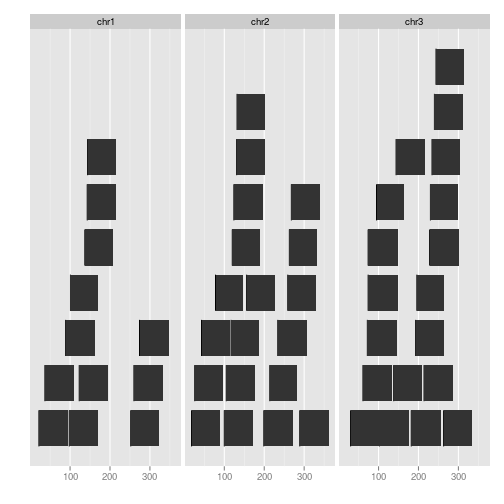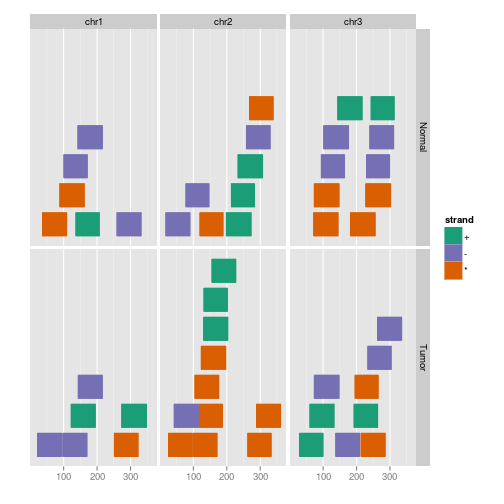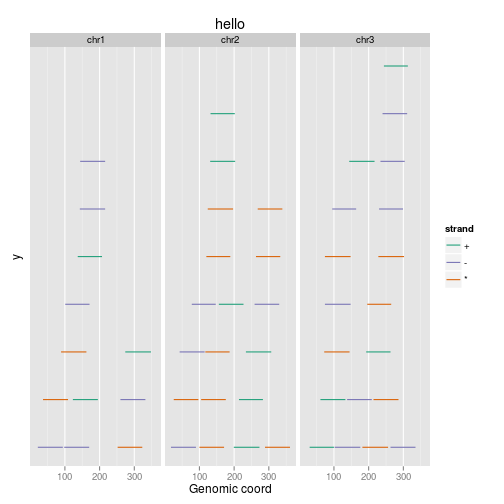stat_stepping {ggbio} R Documentation

## Calculate stepping levels

### Description

Calculate stepping levels.

### Usage

```## S4 method for signature 'GRanges'
stat_stepping(data, ..., xlab, ylab, main,
facets = NULL,
geom = c("rect", "alignment", "segment"))
```

### Arguments

 `data` A `GRanges` or `data.frame` object. `...` Extra parameters such as aes() passed to `geom_rect`, `geom_alignment`, or `geom_segment`. `xlab` Label for x `ylab` Label for y `main` Title for plot. `facets` Faceting formula to use. `geom` The geometric object used to display the data. For 'stepping', could be one of 'rect', 'alignment', 'segment'.

A 'Layer'.

Tengfei Yin

### Examples

``````set.seed(1)
N <- 50
require(GenomicRanges)
##  simul
## ======================================================================
##  simmulated GRanges
## ======================================================================
gr <- GRanges(seqnames =
sample(c("chr1", "chr2", "chr3"),
size = N, replace = TRUE),
IRanges(
start = sample(1:300, size = N, replace = TRUE),
width = sample(70:75, size = N,replace = TRUE)),
strand = sample(c("+", "-", "*"), size = N,
replace = TRUE),
value = rnorm(N, 10, 3), score = rnorm(N, 100, 30),
sample = sample(c("Normal", "Tumor"),
size = N, replace = TRUE),
pair = sample(letters, size = N,
replace = TRUE))
##  default
ggplot(gr) + stat_stepping()
````````````## or
ggplot() + stat_stepping(gr)
````````````##  facet_aes
ggplot(gr) + stat_stepping(aes(color = strand, fill = strand),
facets = sample ~ seqnames)
````````````##  geom_segment
ggplot(gr) + stat_stepping(aes(color = strand),
geom = "segment", xlab = "Genomic coord", ylab = "y", main = "hello")
````````````##  geom_alignment
## ggplot(gr) + stat_stepping(geom = "alignment")
##  geom_alignment_group
##  ggplot(gr) + stat_stepping(aes(group = pair),geom = "alignment")
``````

[Package ggbio version 1.5.20 ]